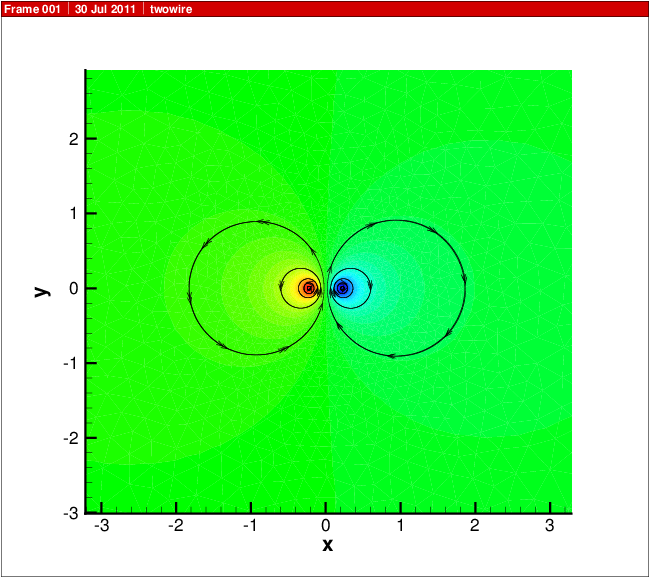# 16. Example Overview¶

In the following chapters, examples are presented for the use of `DEVSIM` to solve some simulation problems. Examples are also located in the `DEVSIM` distribution and their location is mentioned in Directory structure for DEVSIM..

Additional examples are available online, and listed here:

The following example directories are contained in the distribution.

## 16.1. capacitance¶

These are 1D and 2D capacitor simulations, using the internal mesher. A description of these examples is presented in Capacitor.

## 16.2. diode¶

This is a collection of 1D, 2D, and 3D diode structures using the internal mesher, as well as `Gmsh`. These examples are discussed in Diode.

## 16.3. bioapp1¶

This is a biosensor application.

## 16.4. vector_potential¶

This is a 2D magnetic field simulation solving for the magnetic potential. The simulation script is `vector_potential/twowire.py` A simulation result for two wires conducting current is shown in Fig. 16.1.Fig. 16.1 Simulation result for solving for the magnetic potential and field. The coloring is by the Z component of the magnetic potential, and the stream traces are for components of magnetic field.¶

## 16.5. mobility¶

This is an advanced example using electric field dependendent mobility models.